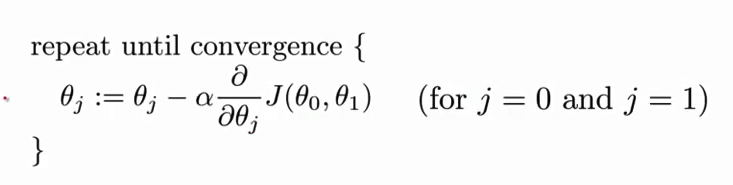## Tuesday, May 13, 2014

### Linear Regression with Multiple Variables using R language

I tried here to derive Linear Regression with Multiple Variables , the same I tried with OCTAVE earlier.

I have a data-set as follows-

 mydata = read.table("D:/tmp/mlclass-ex1-005/mlclass-ex1-005/R-Studio/data.txt",header=TRUE,sep=",")


Now as we know , we should scale our data , as each of the parameters are different in scale. So we will be doing Normalization.

 scale = function(dta,cols,counts){
for(i in 1:counts){
#As we didn't create matrix , the way to fetch column-wise record is as follows-
value = dta[cbind(seq_along(1:nrow(mydata))),i];
sigma = sd(value);
mu = mean(value);
dta[paste(cols[i], ".scale", sep = "")] = (value - mu)/sigma;
#Will append .scale after each processing
}
return (dta);
}where alpha is learning rate which needed to be analysed, so here we'll be running interpretation on some set of alphas-

 alpha = c(0.03, 0.1, 0.3, 1, 1.3, 2,0.4,0.2)


For doing that we need to know about cost Function
What is Cost Function
The idea is look at how the Cost value J(alpha) drops with the number of iterations, the fastest the drop the better, but if goes up then the alpha value is already too large.

So I derived the formula here-

 # the cost for a given theta
cost = function(x,y,th,m) {
prt = ((x %*% t(th)) - y)
return(1/m * (t(prt) %*% prt))
}


Once we done with above we are now ready for running the

Now deriving the Vectorization formula, predict the delta as follows-

 # the delta updates
delta = function(x,y,th) {
delta = (t(x) %*% ((x %*% t(th)) - y))
return(t(delta))
}


Now run each of the alpha or learning rate on cost function-

 # run J for 50x, on each alpha
for (j in 1:length(alpha)) {
for (i in 1:50) {
J[i,j] = cost(x,y,theta,m) # capture the Cost
theta = theta - alpha[j] * 1/m * delta(x,y,theta)
}
}


Once we are done with cosFunction run , we can plot the graph to get the results-

 # lets have a look
par(mfrow=c(length(alpha)/2,2))
for (j in 1:length(alpha)) {
plot(J[,j], type="l", xlab=paste("alpha", alpha[j]), ylab=expression(J(theta)))
}


I got a graph as follows-

From the above , I derived that 0.4 suits my need the best as the graph converged to minimum the fastest and it was idle after that.

Now for finalizing the learning rate alpha, I must run it until convergence so I estimated it till 50000

 for (i in 1:50000) {
theta = theta - 0.4 * 1/m * delta(x,y,theta)
if (abs(delta(x,y,theta)) < 0.0000001) {
}
}


Now I am able to predict the the value-

 # 2. The predicted price of a house with 2000 square feet and 3 bedrooms.
# Don't forget to scale your features when you make this prediction!
print("Prediction for a house with 2000 square feet and 3 bedrooms:")
#value = dta[cbind(seq_along(1:nrow(mydata))),1];
s <- dta[cbind(seq_along(1:nrow(mydata))),1]; #Size
l <- dta[cbind(seq_along(1:nrow(mydata))),2]; #location
b <- dta[cbind(seq_along(1:nrow(mydata))),3]; #Number of Bedrooms
f <- dta[cbind(seq_along(1:nrow(mydata))),4]; #Floor Number
print(theta %*% c(1, (2000 - mean(s))/sd(s), (2 - mean(l))/sd(l),(1 - mean(b))/sd(b),(2 - mean(f))/sd(f)))


All the source code , can be found in my GDrive repository as well.